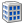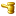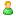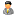##Scientific Potential: Institute of Mathematics and Computer Science

 Science DomainsDDH[+] Physics and mathematics 01.01.01 Mathematical analysis 01.01.02 Differential equations 3 01.01.04 Geometry and topology 1 01.01.06 Mathematical logics, algebra and theory of numbers 1 7 01.01.09 Mathematical cybernetics and operation research 1 01.05.01 Theoretical foundation of computer science; programming 1 5 01.05.04 Mathematical modelling, mathematical methods, software 1 2 [+] Economics 08.00.13 Economico-Mathematical Methods 1